# Sample Data: Murchison Gold Deposits

Locations of gold deposits in Murchison area of Western Australia

## Details

Locations of gold deposits in Murchison area of Western Australia, in the observation region Rectangle[{352783., 6699742}, {682590., 7101484}] meters, without annotations.

## Examples

### Basic Examples (1)

 In:=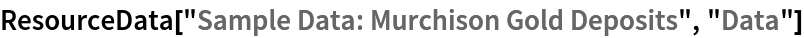Out=Summary of the spatial point data:

 In:=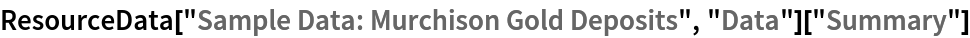Out=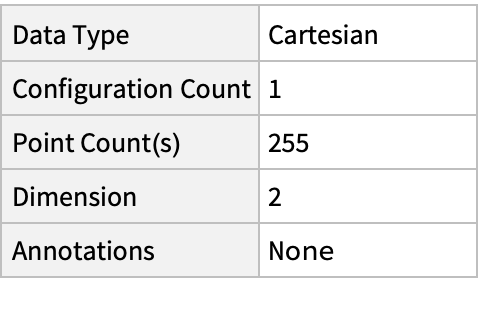### Visualizations (2)

Plot the spatial point data:

 In:=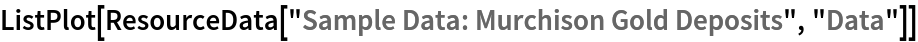Out=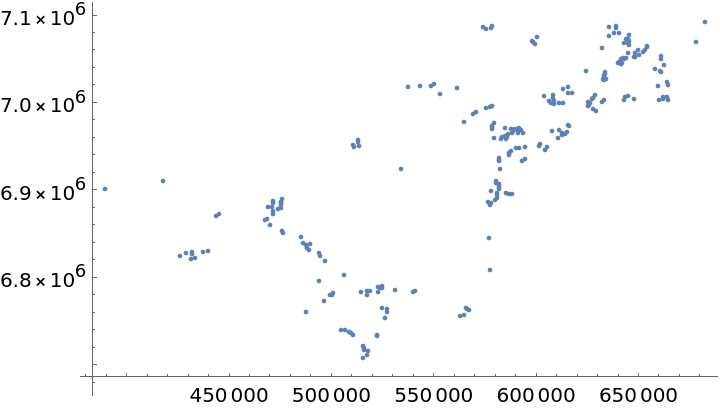Visualize smooth point density:

 In:=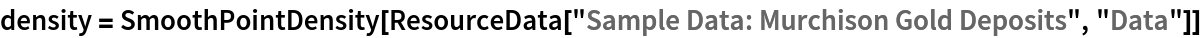Out=In:=Out=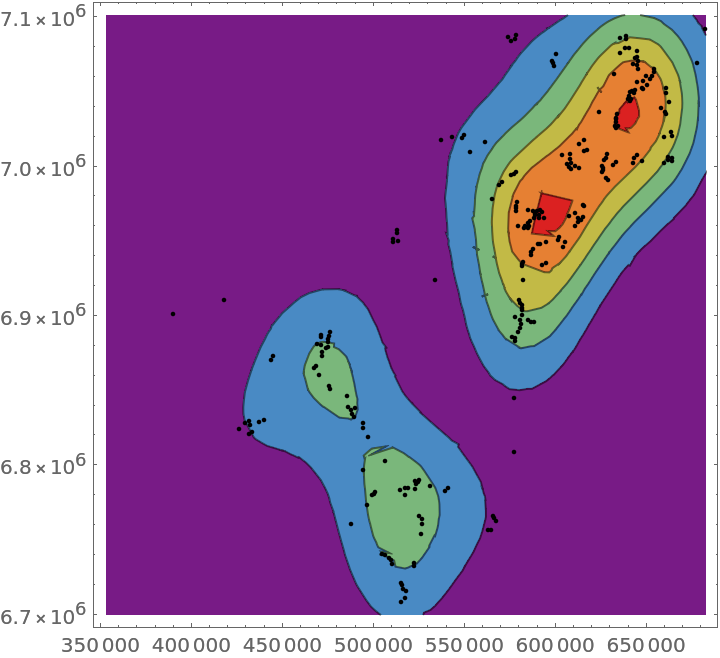### Analysis (3)

Compute probability of finding a point within given radius of an existing point - NearestNeighborG is the CDF of the nearest neighbor distribution:

 In:=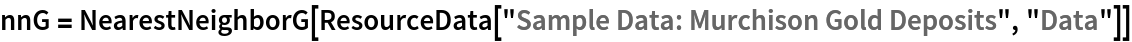Out=In:=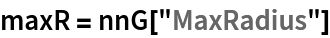Out=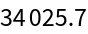In:=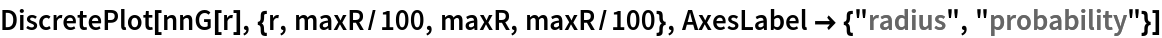Out=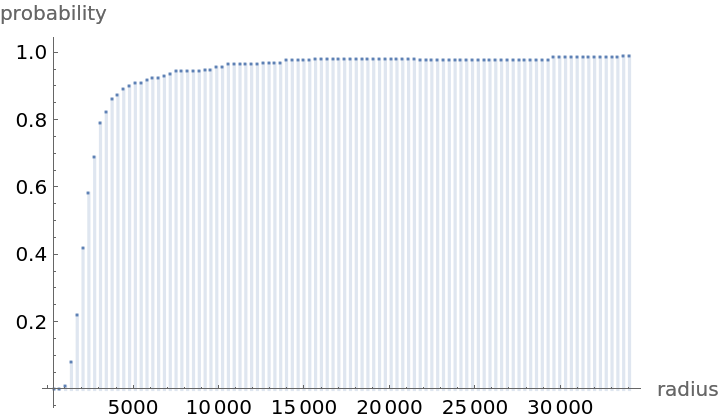Mean distance between a typical point and its nearest neighbor (for positive support distribution can be approximated via a Riemann sum of 1-CDF):

 In:=In:=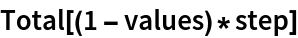Out=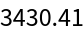Test for complete spacial randomness:

 In:=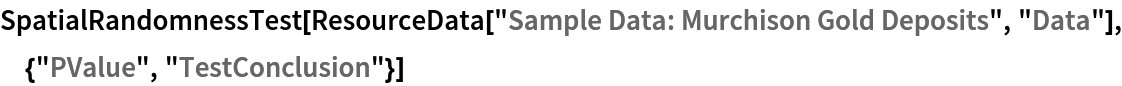Out=Gosia Konwerska, "Sample Data: Murchison Gold Deposits" from the Wolfram Data Repository (2021)